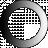# American Dictionary of the English Language

Dictionary Search

### Equation

EQUA'TION, noun [Latin oequatio, from oequo, to make equal or level.]

1. Literally, a making equal, or an equal division.

2. In algebra, a proposition asserting the equality of two quantities, and expressed by the sign=between them; or an expression of the same quantity in two dissimilar terms, but of equal value, as 3s=36d, or x=b+m-r. In the latter case, x is equal to be added to m, with r subtracted, and the quantities on the right hand of the sign of equation are said to be the value of x on the left hand.

3. In astronomy, the reduction of the apparent time or motion of the sun to equable, mean or true time.

4. The reduction of any extremes to a mean proportion.